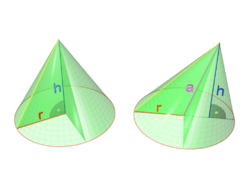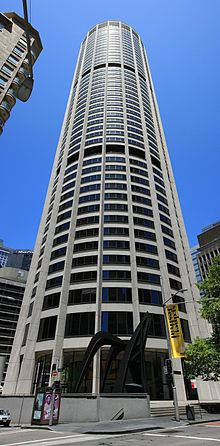# Cone (geometry)

﻿
Cone (geometry)A right circular cone and an oblique circular cone

A cone is an n-dimensional geometric shape that tapers smoothly from a base (usually flat and circular) to a point called the apex or vertex. Formally, it is the solid figure formed by the locus of all straight line segments that join the apex to the base. The term "cone" is sometimes used to refer to the surface or the lateral surface of this solid figure (the lateral surface of a cone is equal to the surface minus the base).

The axis of a cone is the straight line (if any), passing through the apex, about which the base has a rotational symmetry.

In common usage in elementary geometry, cones are assumed to be right circular, where right means that the axis passes through the centre of the base (suitably defined) at right angles to its plane, and circular means that the base is a circle. Contrasted with right cones are oblique cones, in which the axis does not pass perpendicularly through the centre of the base. In general, however, the base may be any shape, and the apex may lie anywhere (though it is often assumed that the base is bounded and has nonzero area, and that the apex lies outside the plane of the base). For example, a pyramid is technically a cone with a polygonal base.

## Other mathematical meanings

In mathematical usage, the word "cone" is used also for an infinite cone, the union of a set of half-lines that start at a common apex point and go through a base. Observe that an infinite cone is not bounded by its base and extends to infinity. A doubly infinite cone, or double cone, is the union of a set of straight lines that pass through a common apex point and go through a base, therefore double infinite cones extend symmetrically on both sides of the apex.

The boundary of an infinite or doubly infinite cone is a conical surface, and the intersection of a plane with this surface is a conic section. For infinite cones, the word axis again usually refers to the axis of rotational symmetry (if any). Either half of a double cone on one side of the apex is called a nappe.

Depending on the context, "cone" may also mean specifically a convex cone or a projective cone.

Also, a oblique shaped cone is known as a circular based pyramid due to the inwardly converging hypos towards the apex point.

## Further terminology

The perimeter of the base of a cone is called the directrix, and each of the line segments between the directrix and apex is a generatrix of the lateral surface. (For the connection between this sense of the term "directrix" and the directrix of a conic section, see Dandelin spheres.)

The base radius of a circular cone is the radius of its base; often this is simply called the radius of the cone. The aperture of a right circular cone is the maximum angle between two generatrix lines; if the generatrix makes an angle θ to the axis, the aperture is 2θ.

A cone with its apex cut off by a plane is called a "truncated cone"; if the truncation plane is parallel to the cone's base, it is called a frustum. An elliptical cone is a cone with an elliptical base. A generalized cone is the surface created by the set of lines passing through a vertex and every point on a boundary (also see visual hull).

## Geometry

### Surface Area

The lateral surface area of a right circular cone is$\!LSA = \pi r l$ where$\!r$ is the radius of the circle at the bottom of the cone and$\!l$ is the lateral height of the cone (given by the Pythagorean theorem$\!l=\sqrt{r^2+h^2}$ where$\!h$ is the height of the cone). The surface area of the bottom circle of a cone is the same as for any circle,$\!\pi r^2$. Thus the total surface area of a right circular cone is:$\!SA=\pi r^2+\pi r l$ or$\!SA=\pi r(r+l)$

### Volume

The volume V of any conic solid is one third of the product of the area B of the base and the height H (the perpendicular distance from the base to the apex).$\!V = \frac{1}{3} B H$

In modern math, this formula can easily be computed using calculus – it is, up to scaling, the integral$\!\int x^2 dx = \tfrac{1}{3} x^3.$ Without using calculus, the formula can be proven by comparing the cone to a pyramid and applying Cavalieri's principle – specifically, comparing the cone to a (vertically scaled) right square pyramid, which forms one third of a cube. This formula cannot be proven without using such infinitesimal arguments – unlike the 2-dimensional formulae for polyhedral area, though similar to the area of the circle – and hence admitted less rigorous proofs before the advent of calculus, with the ancient Greeks using the method of exhaustion. This is essentially the content of Hilbert's third problem – more precisely, not all polyhedral pyramids are scissors congruent (can be cut apart into finite pieces and rearranged into the other), and thus volume cannot be computed purely by using a decomposition argument.

### Center of mass

The center of mass of a conic solid of uniform density lies one-quarter of the way from the center of the base to the vertex, on the straight line joining the two.

### Right circular cone

For a circular cone with radius R and height H, the formula for volume becomes$V = \int_0^H r^2 \pi dh$

where r is the radius of the cone at height h:$r= R \frac{h}{H}$

Thus:$V = \int_0^H [R \frac{h}{H}]^2 \pi dh$

Thus:$V = \frac{1}{3} \pi R^2 H.$

For a right circular cone, the surface area A is$A =\pi R^2 + \pi R S\,$   where$S = \sqrt{R^2 + H^2}$   is the slant height.

The first term in the area formula, πR2, is the area of the base, while the second term, πRS, is the area of the lateral surface.

A right circular cone with height h and aperture , whose axis is the z coordinate axis and whose apex is the origin, is described parametrically as$F(s,t,u) = \left(u \tan s \cos t, u \tan s \sin t, u \right)$

where s,t,u range over [0,θ), [0,2π), and [0,h], respectively.

In implicit form, the same solid is defined by the inequalities$\{ F(x,y,z) \leq 0, z\geq 0, z\leq h\}$,

where$F(x,y,z) = (x^2 + y^2)(\cos\theta)^2 - z^2 (\sin \theta)^2.\,$

More generally, a right circular cone with vertex at the origin, axis parallel to the vector d, and aperture , is given by the implicit vector equation F(u) = 0 where$F(u) = (u \cdot d)^2 - (d \cdot d) (u \cdot u) (\cos \theta)^2$   or$F(u) = u \cdot d - |d| |u| \cos \theta$

where u = (x,y,z), and$u \cdot d$ denotes the dot product.

## Projective geometryIn projective geometry, a cylinder is simply a cone whose apex is at infinity, which corresponds visually to a cylinder in perspective appearing to be a cone towards the sky.

In projective geometry, a cylinder is simply a cone whose apex is at infinity. Intuitively, if one keeps the base fixed and takes the limit as the apex goes to infinity, one obtains a cylinder, the angle of the side increasing as arctan, in the limit forming a right angle.

This is useful in the definition of degenerate conics, which require considering the cylindrical conics.

Wikimedia Foundation. 2010.

### Look at other dictionaries:

• Cone (geometry)/Proofs — Volume * Claim: The volume of a conic solid whose base has area b and whose height is h is {1over 3} b h . Proof: Let vec alpha (t) be a simple planar loop in mathbb{R}^3 . Let vec v be the vertex point, outside of the plane of vec alpha .Let the …   Wikipedia

• Cone — This disambiguation page lists articles associated with the same title. If an internal link led you here, you may wish to change the link to point directly to the intended article …   Wikipedia

• Cone (linear algebra) — In linear algebra, a (linear) cone is a subset of a vector space that is closed under multiplication by positive scalars. In other words, a subset C of a real vector space V is a cone if and only if λx belongs to C for any x in C and any positive …   Wikipedia

• Cone (topology) — Cone of a circle. The original space is in blue, and the collapsed end point is in green. In topology, especially algebraic topology, the cone CX of a topological space X is the quotient space: of the …   Wikipedia

• cone´less — cone «kohn», noun, verb, coned, con|ing. –n. 1. a solid object that has a flat, round base and narrows to a point at the top. 2. Geometry. a surface traced by a moving straight line, one point of which is fixed, the opposite end constantly… …   Useful english dictionary

• Geometry — Ge*om e*try, n.; pl. {Geometries}[F. g[ e]om[ e]trie, L. geometria, fr. Gr. ?, fr. ? to measure land; ge a, gh^, the earth + ? to measure. So called because one of its earliest and most important applications was to the measurement of the earth s …   The Collaborative International Dictionary of English

• Cone tracing — is a derivative of the ray tracing algorithm that replaces rays, which have no thickness, with cones. Cone tracing is related to beam tracing, but uses circular rather than polygonal cross sections. Cone tracing solves certain problems related to …   Wikipedia

• geometry — /jee om i tree/, n. 1. the branch of mathematics that deals with the deduction of the properties, measurement, and relationships of points, lines, angles, and figures in space from their defining conditions by means of certain assumed properties… …   Universalium

• Cone of curves — In mathematics, the cone of toot (sometimes the Kleiman Mori cone) of an algebraic variety X is a combinatorial invariant of much importance to the birational geometry of X. Contents 1 Definition 2 Applications 3 …   Wikipedia

• cone — /koʊn / (say kohn) noun 1. a solid with a circular base, whose straight sides converge in a point. 2. Geometry a solid whose surface is generated by the straight lines joining a fixed point to the points of a plane curve whose plane does not… …   Australian English dictionary